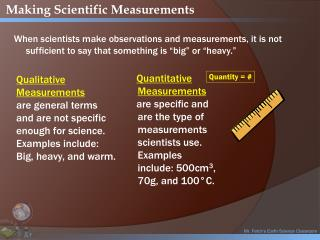DownloadDownload PresentationMr. Fetch’s Earth Science Classroom

# Mr. Fetch’s Earth Science Classroom

Télécharger la présentation## Mr. Fetch’s Earth Science Classroom

- - - - - - - - - - - - - - - - - - - - - - - - - - - E N D - - - - - - - - - - - - - - - - - - - - - - - - - - -
##### Presentation Transcript

1. Making Scientific Measurements When scientists make observations and measurements, it is not sufficient to say that something is “big” or “heavy.” Quantitative Measurements are specific and are the type of measurements scientists use. Examples include: 500cm3, 70g, and 100°C. Quantity= # Qualitative Measurements are general terms and are not specific enough for science. Examples include: Big, heavy, and warm. Mr. Fetch's Earth Science Class Mr. Fetch’s Earth Science Classroom A+

2. Making Scientific Measurements • Scientists use a standard system of measurement know as the: International System of Units (SI system). • The SI system is based on multiples of 10. • Each unit is 10 times larger than the next smallest unit. Mr. Fetch's Earth Science Class Mr. Fetch’s Earth Science Classroom A+

3. Length (distance) • Length is the: distance between two points. • FACTS ABOUT LENGTH MEASURMENTS: • The unit for distance is the meter (m). • Small distances measured in centimeters (cm) or millimeters (mm) • Large distances (here to Richmond) measured in kilometers (km) 1 km= 1000 m Common Conversions 1 m = 100 cm 1 m = 1,000 mm 1 cm = 10 mm Mr. Fetch's Earth Science Class Mr. Fetch’s Earth Science Classroom A+

4. 1 centimeter = 10 millimeters What is the length of the line in centimeters? _______cm What is the length of the line in millimeters? _______mm What is the length of the line to the nearest centimeter? ________cm HINT: Round to the nearest centimeter – no decimals. Measuring Length How many millimeters are in 1 centimeter? Ruler: http://www.k12math.com/math-concepts/measurement/ruler-cm.jpg

5. Area • Area is the: size of a region or space. • FACTS ABOUT AREA MEASURMENTS: • Area is found by multiplying two distances. • Area =(Length) x (Width) • Units are also multiplied and become “squared” 2 Length = 20m Width = 20m (20m) X (20m) = 400m2 20 m 20 m Mr. Fetch's Earth Science Class Mr. Fetch’s Earth Science Classroom A+

6. Volume (square objects, like a box) • Volume is the: amount of space something takes up. • FACTS ABOUT VOLUME MEASURMENTS: • Volume is found by multiplying three distances. • Volume =(Length) x (Width) x (Height) • Units are also multiplied and become “cubed” 3 Length = 10m Width = 10m Height = 20m (10m) X (10m) X (20m) = 2000m3 20 m 10 m 10 m Mr. Fetch's Earth Science Class Mr. Fetch’s Earth Science Classroom A+

7. 50 40 30 20 0 Volume (liquid, like water in a cup) • FACTS ABOUT LIQUID VOLUME MEASURMENTS: • The unit for liquid volume is the Liter (L). • Volume is found using a graduated cylinder. • Units for small volumes are milliliters (ml). You should always read a graduated cylinder at the bottom of the curve of the liquid. That curve is called the meniscus. 1 L= 1000 mLCommon Conversions Mr. Fetch's Earth Science Class Mr. Fetch’s Earth Science Classroom A+

8. 9 cm 8 cm 10 cm We can measure the volume of irregular object using water displacement. Amount of H2O with object = ______About of H2O without object = ______Difference = Volume = ______ http://resources.edb.gov.hk/~s1sci/R_S1Science/sp/en/syllabus/unit14/new/testingmain1.htm Measuring Solid Volume We can measure the volume of regular object using the formula length x width x height. _____ X _____ X _____ = _____ Click here for an online activity about volume. Choose Lessons  Volume & Displacement

9. Mass • Mass is the: amount of matter “stuff” something is made of.Mass will not change unless you add or take away matter. • FACTS ABOUT MASS MEASURMENTS: • The unit for mass is the gram (g). • Large masses are measured in kilograms (kg). • A triple beam balance (NOT A SCALE) is used to measure mass. 1 kg= 1000 gCommon Conversions Mr. Fetch's Earth Science Class Mr. Fetch’s Earth Science Classroom A+

10. Warm upFriday August 31st • Turn in your Earth Layers/ Spheres poster to the tray • Finish your experimental design assignment. Hold on to it for now • Prepare to take the Scientific Methods vocabulary quiz

11. Warm upTuesday September 4th • Complete Scientific Method Wordsearch • 3 students with most words made out of the letters in “Scientific Method” wins a ticket